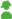#72 – The Rule of 72

By SoGiAm, April 10, 2016

Rule Of 72 according to Investopedia:
What is the ’Rule Of 72’
The rule of 72 is a shortcut to estimate the number of years required to double your money at a given annual rate of return. The rule states that you divide the rate, expressed as a percentage, into 72:

Years required to double investment = 72 ÷ compound annual interest rate

Note that a compound annual return of 8% is plugged into this equation as 8, not 0.08, giving a result of 9 years (not 900).

Next Up
COMPOUND INTEREST
ANNUAL PERCENTAGE YIELD – APY
COMPOUNDING
RETURN
BREAKING DOWN ’Rule Of 72’
The rule of 72 is a useful shortcut, since the equations related to compound interest are too complicated for most people to do without a calculator. To find out exactly how long it would take to double an investment that returns 8% annually, one would have to use this equation:

T = ln(2)/ln(1.08)=9.006

Most people cannot do logarithmic functions in their heads, but they can do 72 ÷ 8 and get almost the same result. Conveniently, 72 is divisible by 2, 3, 4, 6, 8, 9, and 12, making the calculation even simpler.

The rule can also be used to find the amount of time it takes for money’s value to halve due to inflation. If inflation is 6%, then a given amount of money will be worth half as much in 72 ÷ 6 = 12 years. Nor does the unit have to be money: the rule could apply to population, for example.

The rule of 72 is reasonably accurate for interest rates between 6% and 10%. When dealing with rates outside this range, the rule can be adjusted by adding or subtracting 1 from 72 for every 3 points the interest rate diverges from 8%. So for 11% annual compounding interest, the rule of 73 is more appropriate; for 14%, it would be the rule of 74; for 5%, the rule of 71.

For example, say you have a 22% rate of return (congratulations). The rule of 72 says the initial investment will double in 3.27 years. Since 22 – 8 is 14, and 14 ÷ 3 is 4.67 ≈ 5, the adjusted rule would use 72 + 5 = 77 for the numerator. This gives a return of 3.5, meaning you’ll have to wait another quarter to double your money. The period given by the logarithmic equation is 3.49, so the adjusted rule is more accurate.